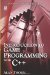# 7.4 Conditional Statements

## 7.4 Conditional Statements

Statements that use conditional operators to compare quantities are called conditional statements. For example, A>Bisa conditional statement. It compares the values of A and B to see if one is larger than the other. Conditional statements evaluate to Boolean values, that is, either true or false, 1 or 0. So, if A really is greater than B, then (A > B) = true. In other words, (A > B) is substituted by true. Consider the sample code below:

`      int Variable_1 = 5;      int Variable_2 = 11;      char Letter = 'Y';      bool Result1 = (9 > 7);      bool Result2 = (7 == Variable_1);      bool Result3 = (Variable_1 != Variable_2);      bool Result4 = (Letter == 'Y'); `

Here, a number of Boolean variables are created, namely Result1 through Result4. Each variable will be either true or false, reflecting the truth of the Boolean statement assigned to the variable on the other side of the equal sign. So, Result1 is true because 9 is greater than 7, and Result2 is false because variable_1 is not equal to 7. Result3 is true because variable_1 is not equal to variable_2, and Result4 is true because the variable Letter is equal to "Y".

### 7.4.1 If Statement

Based upon whether a conditional statement is true or false, a program may need to respond in different ways. For example, the user has been asked whether the program is to terminate and is expected to press either Y to terminate or N to continue. The program needs to determine which key was pressed and how to respond. It should end if Y is pressed and continue if N is pressed. To handle such decision making, the If statement can be used. The if keyword causes a program to branch off and execute a specific set of instructions only if a statement is true. It is equivalent to human reasoning, such as: "If the pressed key is Y, then terminate the program." From the previous sentence, the statement "The pressed key is Y" is a conditional statement; it's true if the pressed key is Y and false if it's any other key. The If statement takes the following form:

• if (condition == true) {do something}

### 7.4.2 Using If

As mentioned, a program enters a specific branch using the If statement when, and only when, a statement is true. Consider the following code:

`      #include <iostream>      int main()      {         char Key = 'N';         std::cin >> Key;         if(Key == 'Y')         {         std::cout<<"Y was pressed\n";         }         return 0;      } `

The code above demonstrates how if works. The keyword if is followed by a condition that must be true for the If statement to continue. If true, the branch of code collected inside the braces is executed. If the condition is false, the code inside the braces is ignored, and processing continues after the braces.

### 7.4.3 If Else Statement

Following naturally from the If statement is an extension called the If Else statement. Whereas the If statement caused the program to enter a specific branch of execution only when a given condition was true, the If Else causes a program to branch off into one of two possible branches, depending on whether the condition is true or false. If true, the program enters one branch; if it's false, the program enters another. Like the If statement, the If Else has an equivalent human reasoning: "If X is true, then do this; else do this." If Else has the following form:

`      if(condition==true) {do something;}          else {do something;} `

### 7.4.4 Using If Else

A program can enter one of two branches using If Else; one branch if the condition is true, and another if it's false. This is demonstrated in the following C++ code sample:

`      #include <iostream>      int main()      {         char Key = 'N';         std::cin >> Key;         if(Key == 'Y')         {            std::cout<<"Y was pressed\n";         }         else         {            std::cout<<"Y was not pressed\n";         }         return 0;      } `Introduction to Game Programming with C++ (Wordware Game Developers Library)
ISBN: 1598220322
EAN: 2147483647
Year: 2007
Pages: 225
Authors: Alan Thorn

Similar book on Amazon

flylib.com © 2008-2017.
If you may any questions please contact us: flylib@qtcs.net